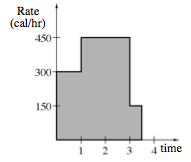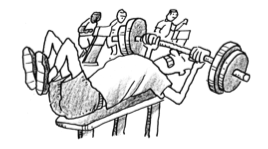### Home > CALC > Chapter 2 > Lesson 2.1.2 > Problem2-21

2-21.
1. William wants to find out how many calories he burns while at the gym. The number of calories he burns depends on which exercises he does during his workout. Given the graph below of the rate he burns calories, answer the questions below. Homework Help ✎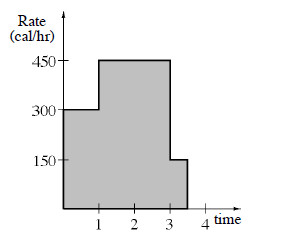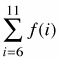1. How many calories does William burn during the first hour of exercise? Support your answer with a short explanation of how you arrived at this result.

2. How many calories does William burn by the end of his workout?

3. What is the average number of calories that he burns per hour?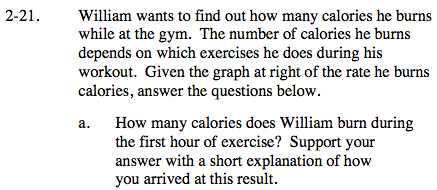Since area of rectangles = (base)(height)on this graph: area = (hours)(calories/hour) = calories. So compute the area under the curve between t = 0 and t = 1.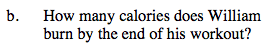Refer to the hint for part (a).

In order to use known geometric formulas, you will have to dissect this problem into parts.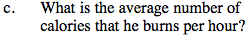$\text{Average calories }= \frac{\text{total calories}}{\text{total time}}$